Learning Division Facts Book: You Pick Numbers to Focus On
Perfect for learning Division - especially the first page!

Division Chart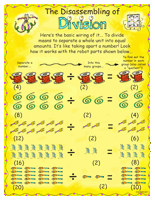Disassembling Division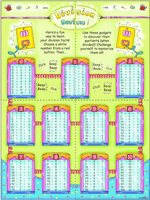Division Devices (Large Chart)

Runaway Math Puzzles
 Runaway Math PuzzlesDivision Math Center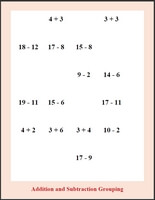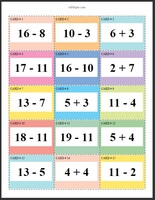Division Math Center: Numbers 2 to 9 (Grades 3-6) Division Math Center: Numbers 2 to 12 (Grades 3-6)

Multiplication and Division Math CenterMultiplication and Division Math Center: Numbers 2 to 9 (Grades 3-6) Multiplication and Division Math Center: Numbers 2 to 12 (Grades 3-6)

Math Facts

Quick Math Facts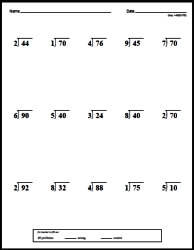Make Quick Math Facts Printable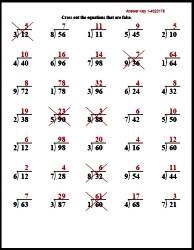Color by Division

Introduction to Division
Division Concepts (with graphics of pictures)
Divide into groups (smaller numbers)
Divide into groups (larger numbers)
Divide into groups (mix)
Division Concepts with left over graphics
Divide into groups (smaller numbers)
Divide into groups (larger numbers)
Divide into groups (mix)
Division Facts (with graphics)
Write a division fact (smaller numbers)
Write a division fact (larger numbers)
Write a division fact (mix)
Multiplication and Division
Fill in the quotient
Fill in the missing number
Fill in the missing number
Division Problem Solving
Division problem solving
Division Mixed Review
Introduction to Division Mixed Review

Division Facts Through 10
Multiplication and Division: Fill in the missing numbers
Multiplication and division with 2
Multiplication and division with 3
Multiplication and division with 4
Multiplication and division with 5
Multiplication and division with 6
Multiplication and division with 7
Multiplication and division with 8
Multiplication and division with 9
Multiplication and division with 10
Multiplication and division (mix)
Division Facts
Divide by 2
Divide by 3
Divide by 4
Divide by 5
Divide by 6
Divide by 7
Divide by 8
Divide by 9
Divide by 10
Division (mix)
Division Facts
Division by 2 to 10
Division by 2 to 10
Fill in the missing number
Division Problem Solving
Division problem solving
Division Mixed Review
Division Facts Mixed Review

Easy Division Activity Books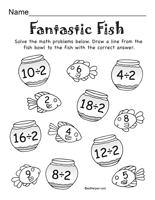Division Activity Pages (book #1)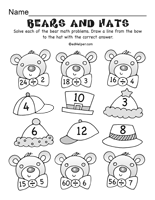Division Activity Pages (book #2)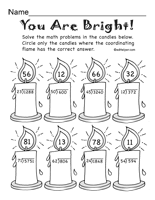Division Activity Pages (book #3)

Division Word Problems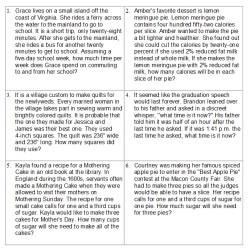Division Word Problems (3rd grade) Division Word Problems (4th grade) Division Word Problems (5th grade) Division Word Problems (6th grade)

Division by One-Digit Numbers
Column Division with Extra Space To Solve Problems - Division by One-Digit Numbers
2 digits by 1 digit column division (no remainders)
2-3 digits by 1 digit column division (no remainders)
4-5 digits by 1 digit column division (no remainders)
5-7 digits by 1 digit column division (no remainders)
2 digits by 1 digit column division
2-3 digits by 1 digit column division
4-5 digits by 1 digit column division
5-7 digits by 1 digit column division
2 digits (and increasing 1 digit every 2 rows) by 1 digit column division
2 digits (and increasing 1 digit every row) by 1 digit column division

Column Division (more problems with less space) - Division by One-Digit Numbers
2 digits by 1 digit column division (no remainders)
2-3 digits by 1 digit column division (no remainders)
4-5 digits by 1 digit column division (no remainders)
5-7 digits by 1 digit column division (no remainders)
2 digits by 1 digit column division
2-3 digits by 1 digit column division
4-5 digits by 1 digit column division
5-7 digits by 1 digit column division
2 digits (and increasing 1 digit every 2 rows) by 1 digit column division
2 digits (and increasing 1 digit every row) by 1 digit column division

Part 2 - Division by One-Digit Numbers
Divisibility: complete the table
2 digits by 1 digit across division
2-3 digits by 1 digit across division
4-5 digits by 1 digit across division
2 digits (and increase by 1 digit every row) by 1 digit across division
Fill in the missing numbers: 2 digits by 1 digit
Fill in the missing numbers: 3 digits by 1 digit
Fill in the missing numbers: 4 digits by 1 digit
Division Word Problems
Division Word Problems
Solve equations: 2 digits by 1 digit
Order of operations: 2-3 digits by 1 digit (no parenthesis)
Order of operations: 2-3 digits by 1 digit
Order of operations: 2-4 digits by 1 digit
One-Digit Numbers Division Mixed Review

Division by Two-Digit Numbers
Column Division with Extra Space To Solve Problems - Division by Two-Digit Numbers
Division by multiples of 10: 3-4 digits by 2 digits (no remainders)
Division by multiples of 10: 5-7 digits by 2 digits (no remainders)
Division by multiples of 10: 3-4 digits by 2 digits
3-4 digits by 2 digits column division (no remainders)
4-5 digits by 2 digits column division (no remainders)
5-6 digits by 2 digits column division (no remainders)
6-7 digits by 2 digits column division (no remainders)
3-4 digits by 2 digits column division
4-5 digits by 2 digits column division
5-6 digits by 2 digits column division
6-7 digits by 2 digits column division
3 digits (and increasing 1 digit every 2 rows) by 2 digits column division
3 digits (and increasing 1 digit every row) by 2 digits column division

Column Division (more problems with less space) - Division by Two-Digit Numbers
Division by multiples of 10: 3-4 digits by 2 digits (no remainders)
Division by multiples of 10: 5-7 digits by 2 digits (no remainders)
Division by multiples of 10: 3-4 digits by 2 digits
3-4 digits by 2 digits column division (no remainders)
4-5 digits by 2 digits column division (no remainders)
5-6 digits by 2 digits column division (no remainders)
6-7 digits by 2 digits column division (no remainders)
3-4 digits by 2 digits column division
4-5 digits by 2 digits column division
5-6 digits by 2 digits column division
6-7 digits by 2 digits column division
3 digits (and increasing 1 digit every 2 rows) by 2 digits column division
3 digits (and increasing 1 digit every row) by 2 digits column division

Part 2 - Division by Two-Digit Numbers
Divisibility: complete the table
3-4 digits by 2 digits across division
4-5 digits by 2 digits across division
5-6 digits by 2 digits across division
3 digits (and increase by 1 digit every row) by 2 digits across division
Fill in the missing numbers: 3 digits by 2 digits
Fill in the missing numbers: 4 digits by 2 digits
Fill in the missing numbers: 5 digits by 2 digits
Division Word Problems
Division Word Problems
Solve equations: 3 digits by 2 digits
Solve equations: 4-5 digits by 2 digits
Order of operations: 2-3 digits by 1 digit
Order of operations: 3 digits by 2 digits
Two-Digit Numbers Division Mixed Review

Divide Decimals
Extra Space To Solve Problems
Divide a decimal (up to hundredths) by a whole number with no remainders
Divide a decimal (up to thousandths) by a whole number with no remainders
Divide a decimal (up to hundredths) by a whole number with remainders (give the remainder)
Add Zeros: Divide a decimal (up to hundredths) by a whole number with no remainders
Add Zeros: Divide a decimal (up to hundredths) by a whole number with no remainders
Add Zeros: Divide a decimal (up to hundredths) by a whole number with no remainders
Add Zeros: Larger numbers; dividing by a whole number
Divide a whole number by a decimal
Divide a decimal by a decimal (up to tenths)
Divide a decimal by a decimal (up to hundredths)

More Problems with Less Space
Divide a decimal (up to hundredths) by a whole number with no remainders
Divide a decimal (up to thousandths) by a whole number with no remainders
Divide a decimal (up to hundredths) by a whole number with remainders (give the remainder)
Add Zeros: Divide a decimal (up to hundredths) by a whole number with no remainders
Add Zeros: Divide a decimal (up to hundredths) by a whole number with no remainders
Add Zeros: Divide a decimal (up to hundredths) by a whole number with no remainders
Add Zeros: Larger numbers; dividing by a whole number
Divide a whole number by a decimal
Divide a decimal by a decimal (up to tenths)
Divide a decimal by a decimal (up to hundredths)

Part 2
Across division: divide a decimal (up to thousandths) by a whole number with no remainders
Order of Operations (no parenthesis, up to 9.9)
Order of Operations (no parenthesis, up to 99.9)
Order of Operations
Order of Operations (one missing number)
Order of Operations (one missing operation)
Order of Operations (fill in all numbers)
Order of Operations (fill in all operations)
Decimal division word problems
Division of decimals: Mixed Review

Division from Number Word Searches!
Division with numbers 1-6   - with number words    (Fill-In Puzzle)
Division with numbers 1-9   - with number words    (Fill-In Puzzle)
Division with the number one   - with number words    (Fill-In Puzzle)
Division with the number two   - with number words    (Fill-In Puzzle)
Division with the number three   - with number words    (Fill-In Puzzle)
Division with the number four   - with number words    (Fill-In Puzzle)
Division with the number five   - with number words    (Fill-In Puzzle)
Division with the number six   - with number words    (Fill-In Puzzle)
Division with the number seven   - with number words    (Fill-In Puzzle)
Division with the number eight   - with number words    (Fill-In Puzzle)
Division with the number nine   - with number words    (Fill-In Puzzle)
Division with the number ten   - with number words    (Fill-In Puzzle)
Division with the number eleven   - with number words    (Fill-In Puzzle)
Division with numbers 1-13   - with number words    (Fill-In Puzzle)
Division with numbers 10-20   - with number words    (Fill-In Puzzle)
Division with numbers 1-20   - with number words    (Fill-In Puzzle)
2 Digit Division   - with number words    (Fill-In Puzzle)
3 Digit Division   - with number words
2 and 3 Digit Division   - with number words

Division from Missing Digits
Division Missing Digits (2 Digits divided by 1 Digit)
Division Missing Digits (3 Digits divided by 1 Digit)
Division Missing Digits (4 Digits divided by 1 Digit)
Division Missing Digits (3 Digits divided by 2 Digits)
Division Missing Digits (4 Digits divided by 2 Digit)
Division Missing Digits (2-3 Digits divided by 1-2 Digits)
Division Missing Digits (2-4 Digits divided by 1-2 Digits)

Division from Number Puzzles!
* The following worksheets are for edHelper.com subscribers.
Introduction to Division (graphics)
How many 2's are there in 26...
Division (Across Divide by 2 - 12)
Division (Fill in the Missing Factor)
Division (Small Numbers - No Remainders)
Division (Bigger Numbers - No Remainders)
Division (Remainders)
Division (Remainders 3 Digits by 2-12)
Division (No Remainders 3-4 Digits by 2-12)
Division (Remainders 3-4 Digits by 2-12)
Division (mixed remainders and no remainders)
Grouped Division (includes -,+ and 3+ numbers)

Decimals from Number Puzzles!
Multiplication and Division (no remainders)
Multiplication and Division (more difficult - no remainders)
Multiplication and Division (with remainders)

Division Math Money CrossNumber Puzzles from Number Puzzles!
Money Multiplication (Number Puzzle)
Money Division (Number Puzzle)
Money Multiplication and Division (Number Puzzle)
Money Addition, Subtraction, Multiplication and Division (Number Puzzle)

Fractions from Number Puzzles!
Division
Division (Mixed Fractions)
Multiplication and Division

Integers from Number Puzzles!
Division
Multiplication and Division
Mixed
Multiplication and Division (more...)

Division Sequences from Sequences Puzzles!
Division Sequences

Multiplication of Fractions from Fraction Worksheets!
Division
Division (Mixed Fractions)
Multiplication and Division

EdHelper Subscriber Only Materials
* This section is for edHelper.com subscribers. A subscription to edHelper includes access to the math, spelling, and vocabulary subscription areas. Sign up for the subscriber materials.

Time: TV (Includes Fraction Division)

Build Worksheet - 3 People, 4 People, 5 People, 6 People, 7 People

Division Word Problems from the Math Standardized Test Prep Section
* This section is for edHelper.com subscribers. A subscription to edHelper includes access to the math, spelling, and vocabulary subscription areas. Sign up for the subscriber materials.

 Division Sheet #1 Sheet #2 Sheet #3 Sheet #4 Sheet #5 Review Sheet

 HarderDivision Sheet #1 Sheet #2 Sheet #3 Sheet #4 Review Sheet

More Activities and Printables
 Divisions

Have a suggestion or would like to leave feedback?